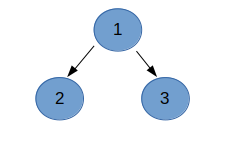Minimize the value
Tag(s):

Algorithms, Breadth-first search, Depth First Search, Depth-first search, Easy, Graph, Graph Theory

Problem
Editorial
Analytics

You are given a binary tree rooted at 1. Initially, all the nodes of the tree have some initial values Vi. Wave operation is to be applied on the tree.

After applying the wave operation,
Value of each node in the tree = Sum of all initial values of nodes in its subtree.

You are required to add 1 more node with value to the tree such that:
1. The tree remains binary after adding the node to the tree.
2. After applying the wave operation to this tree (the tree after adding node with value X), the sum of tree is minimum.

Sum of tree = sum of values of all nodes in the tree.

Print the minimum sum of the tree.

Input :

• First line of input contains 2 integers and , number of nodes in the tree and value of new node to add respectively.
• Second line contains space separated integers denoting value of each node.
• Each of the following N - 1  lines contains 2 integers and , representing edge between node u and node v.

Output :

• Print the minimum sum possible after adding node with value X and applying wave operation such that tree remains binary tree.

Constraints :

• $1 \le N \le 10^{5}$
• $1 \le V_{i},X \le 10^{9}$
• $1 \le u,v \le N ,\space u \ne v$
SAMPLE INPUT
2 1
1 1
1 2
SAMPLE OUTPUT
5
Explanation

Tree after adding new node (say it 3):Adding node 3 to root node and applying wave operation,

Value of node 1= 1+1+1= 3 (sum of initial value of nodes 1,2 and 3 as they form the subtree of node 1)
Value of node 2 = 1
Value of node 3 = 1
Sum of tree = 3+1+1 = 5 which is the minimum possible sum we can get.

Time Limit: 1.0 sec(s) for each input file.
Memory Limit: 256 MB
Source Limit: 1024 KB
Marking Scheme: Marks are awarded when all the testcases pass.
Allowed Languages: Bash, C, C++, C++14, Clojure, C#, D, Erlang, F#, Go, Groovy, Haskell, Java, Java 8, JavaScript(Rhino), JavaScript(Node.js), Julia, Kotlin, Lisp, Lisp (SBCL), Lua, Objective-C, OCaml, Octave, Pascal, Perl, PHP, Python, Python 3, R(RScript), Racket, Ruby, Rust, Scala, Swift, Swift-4.1, TypeScript, Visual Basic

CODE EDITOR

Initializing Code Editor...

Contributor